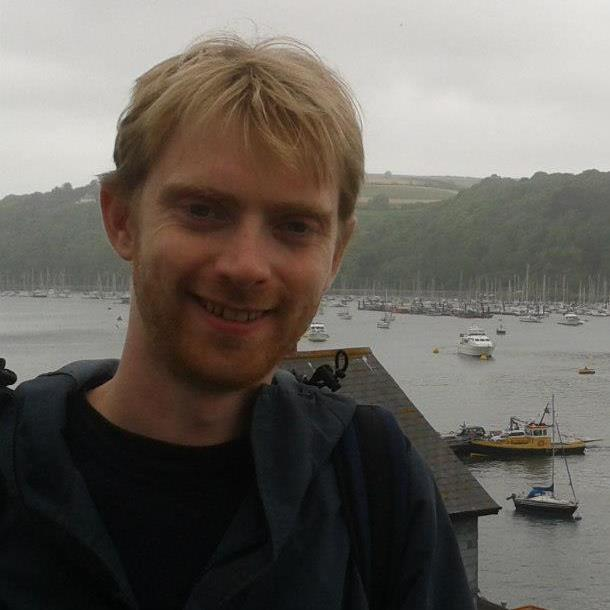# Nicholas Simm## Dr Nicholas Simm

Research Fellow

Office: C2.08
Phone: +44 (0)24 761 50774
Email: n dot simm at warwick dot ac dot uk

Teaching responsibilities 2017/18:

MA901 Fundamental Tools (Shared with statistics.)

I am a Leverhulme Early Career Fellow at the Mathematics Institute, University of Warwick.

I work on mathematical physics and probability - specifically on the theory and applications of random matrices (RMT). I am interested in the issue of Gaussian fluctuations in RMT and its relation to the Gaussian free field or other `log-correlated' stochastic processes. (pre-print 1, publications 3, 5 and 7 below).

My thesis topic concerned the random matrix approach to quantum systems with a chaotic classical limit, mainly on transport properties in these systems and the relation to orthogonal polynomials, integrable hierarchies and Painlevé equations (see publications 9, 10, 11). This is a topic I continue to work on with my collaborators (publications 4 and 6).

Finally, I have worked on `fundamental problems' in RMT - such as `How many eigenvalues of a large random matrix are real (publication 1) and what is the probability distribution of this number?' (publication 3) or 'What is the probability that a random Hermitian matrix is positive definite?' (publication 2).

Pre-prints

1. "Subcritical multiplicative chaos for regularized counting statistics from random matrix theory" (with G. Lambert and D. Ostrovsky) arXiv:1612.02367 (submitted).

Published papers

1. "On the real spectrum of a product of Gaussian random matrices" Electronic Communications in Probability 22, no. 41 (11pp), 2017. (arXiv:1701.09176)
2. "On the probability of positive-definiteness in the gGUE via semi-classical Laguerre polynomials" (with A. Deaño). Journal of Approximation Theory 220, 44-59, 2017. (arXiv:1610.08561)
3. "Central limit theorems for the real eigenvalues of large Gaussian random matrices". Random Matrices: Theory and Applications 06, 1750002, 2017. (arXiv:1512.01449)
4. Large-N expansion for the time-delay matrix of ballistic chaotic cavities” (with F. D. Cunden, F. Mezzadri and P. Vivo). Journal of Mathematical Physics 57, 111901, 2016. (arXiv:1607.00250)
5. Fractional Brownian Motion with Hurst Index H=0 and the Gaussian Unitary Ensemble” (with Y. V. Fyodorov and B. A. Khoruzhenko). Annals of Probability 44, 4, 2980-3031, 2016. (arXiv:1312.0212)

6. Correlators for the Wigner-Smith time-delay matrix of chaotic cavities” (with F. D. Cunden, F. Mezzadri and P. Vivo). Journal of Physics A: Mathematical and Theoretical 49, 18LT01, 2016. (arXiv:1601.06690)

7. On the distribution of the maximum value of the characteristic polynomial of GUE random matrices” (with Y. V. Fyodorov). Nonlinearity 29, 9, 2016. (arXiv:1503.07110)
8. Energy landscape of the finite-size mean-field 2-spin spherical model and topology trivialization” (with D. Mehta, J. D. Hauenstein, M. Niemerg and D. A. Stariolo). Physical Review E. 91, 022133 2015. (arXiv:1409.8303)
9. Tau-Function Theory of Quantum Chaotic Transport with beta = 1,2,4” (with F. Mezzadri). Communications in Mathematical Physics 324, 465-513, 2013. (arXiv:1206.4584)
10. Moments of the transmission eigenvalues, proper delay times, and random matrix theory II” (with F. Mezzadri). Journal of Mathematical Physics 53 053504, 2012. (arXiv:1108.2859)
11. Moments of the transmission eigenvalues, proper delay times, and random matrix theory I” (with F. Mezzadri). Journal of Mathematical Physics 52 103511, 2011. (arXiv:1103.6203)

Teaching Responsibilities 2015/16:

Term 2: MA254 Theory of ODEs